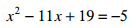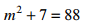#### MAT-HS.A-REI.04

 MAT-HS Targeted Standards(A) Concept: Algebra(REI) Domain: Reasoning with Equations and InequalitiesCluster: Understand solving equations as a process of reasoning and explain the reasoning MAT-HS.A-REI.04  Solve quadratic equations in one variable.   a.  Use the method of completing the square to transform any quadratic equation in x into an equation of the form (x – p)² = q that has the same solutions.   (+) Derive the quadratic formula from this form.   b.  Solve quadratic equations by inspection (e.g., for x² = 49), taking square roots, completing the square, the quadratic formula and factoring, as appropriate to the initial form of the equation. Recognize when the quadratic formula gives complex solutions and write them as a ± bi for real numbers a and b.

## Student Learning Targets:

• I can
• I can

### Reasoning Targets

• I can explain why taking the square root of both sides of an equation can yield two solutions.

### Skills (Performance) Targets

• I can solve for (isolate) a specific variable.
• I can perform transformations that produce equivalent equations (e.g., adding the same amount to both sides of the equation, etc.).
• I can check the solution.
• I can solve for (isolate) a specific variable.
• I can solve a quadratic.
• I can multiply and factor polynomials.
• I can solve quadratic equations in one variable.
• I can transform a quadratic equation to an equation in the form (x-p)2=q by completing the square.
• I can derive the quadratic formula by completing the square on a quadratic equation.
• I can solve quadratic equations in one variable by simple inspection, taking the square root, factoring, and completing the square.
• I can use the quadratic formula to solve any quadratic equation, recognizing the formula produces all complex solutions and write the solutions in the form a ± bi , where a and b are real numbers.

• I can
• I can

## Algebra II Solving Quadratic Equations Proficiency Scale

 Score Description Sample Activity 4.0 In addition to Score 3.0, the student demonstrates in-depth inferences and applications regarding more complex material that go beyond end of instruction expectations. Suppose the quadratic functions f(x)=ax2+bx+c with f(-2)=0 and -b/(2a) =1. Solve f(x)=0. 3.5 In addition to Score 3.0 performance, the student demonstrates in-depth inferences and applications regarding the more complex content with partial success. 3.0 The student can: solve a quadratic equation using any method. Solve the following quadratic equation using any method:x2+4x+15=0 2.5 The student demonstrates no major errors or omissions regarding the simpler details and processes (Score 2.0 content) and partial knowledge of the more complex ideas and processes (Score 3.0 content). 2.0 There are no major errors or omissions regarding the simpler details and processes as the student can: solve simple quadratic equations. recognize and recalls specific terminology, such as: quadratic equation factor complete the square quadratic formula square root imaginary/complex numbers Solve the following quadratic equations:x2+100=0 Perform the given operation: (3+i)(-6-i) 1.5 The student demonstrates partial knowledge of the simpler details and processes (Score 2.0 content) but exhibits major errors or omissions regarding the more complex ideas and procedures (Score 3.0 content). 1.0 With help, the student demonstrates a partial understanding of some of the simpler details and processes (Score 2.0 content) and some of the more complex ideas and processes (Score 3.0 content). - 0.5 With help, the student demonstrates a partial understanding of some of the simpler details and processes (Score 2.0 content) but not the more complex ideas and processes (Score 3.0 content). 0.0 Even with help, the student demonstrates no understanding or skill. -

## Resources

 Web Vocab completing the square quadratic formula